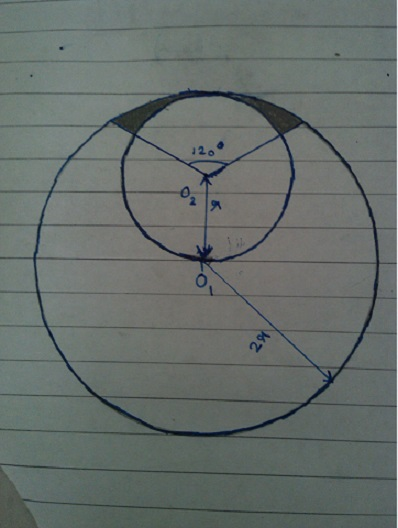# Find the area

Geometry Level 5A circle with centre ${ O }_{ 1 }$ and radius $2r$ is drawn. A point ${ O }_{ 2 }$ is taken at a distance $r$ from ${ O }_{ 1 }$. Now a circle is drawn with centre ${ O }_{ 2 }$ and radius $r$. Now two lines are drawn from ${ O }_{ 2 }$ each making an angle ${ 60 }^{ 0 }$ with ${ O }_{ 1 }$${ O }_{ 2 }$ produced and the given figure is obtained.

If the area of the shaded region in the given figure can be written as $A=k{r}^{2}$ then find $\left\lfloor 100k \right\rfloor$.

Note: The starting part of the question is just a description of how the figure is drawn. The figure must be taken as the reference for finding the shaded region.

×